Courses

# Previous Year Questions (2014-19) - Electrochemistry Notes | EduRev

## NEET : Previous Year Questions (2014-19) - Electrochemistry Notes | EduRev

The document Previous Year Questions (2014-19) - Electrochemistry Notes | EduRev is a part of the NEET Course Chemistry 28 Years Past year papers for NEET/AIPMT Class 12.
All you need of NEET at this link: NEET

Q.1. For a cell involving one electron E°cell = 0.59 V at 298 K, the equilibrium constant for the cell reaction is :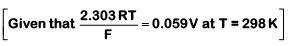(2019)
A: 1.0 × 102
B: 1.0 × 105
C: 1.0 × 1010

D: 1.0 × 1030
Ans:
C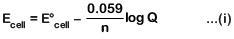(At equilibrium, Q = Keq and Ecell = 0)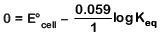(from equation (i))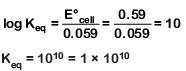Q.2. For the cell reaction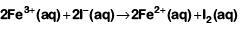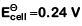at 298 K. The standard Gibbs energy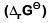of the cell reaction is :[Given that Faraday constant F = 96500 C mol–1]    (2019)
A: – 46.32 kJ mol–1
B: – 23.16 kJ mol–1
C: 46.32 kJ mol–1
D: 23.16 kJ mol–1
Ans:
A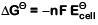= – 2 × 96500 × 0.24 J mol–1
= – 46320 J mol–1
= – 46.32 kJ mol–1

Q.3. Consider the change in oxidation state of Bromine corresponding to different emf values as shown in the diagram below: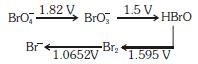Then the species under going disproportionation is:-    (2018)
A: BrO3-
B: BrO4-
C: Br2
D: HBrO
Ans:
D
Calculate E°cell corresponding to each compound under going disproportionation reaction. The reaction for which E°cell comes out +ve is spontaneous.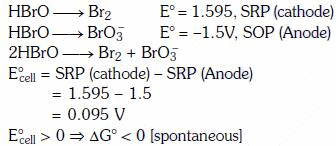Q.4.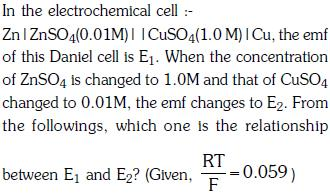(2017)
A: E1 < E2
B: E1 > E2
C: E2 = 0 ≠ E1
D: E1 = E2
Ans:
B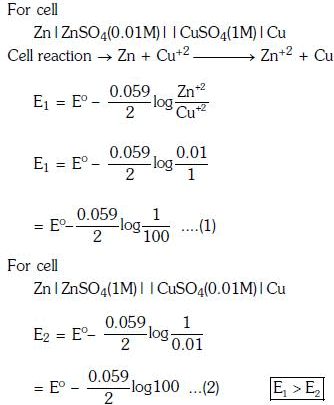Q.5. The pressure of H2 required to make the potential of H2-electrode zero in pure water at 298 K is :    (2016)
A: 10-4 atm
B: 10-14 atm
C: 10-12 atm
D: 10-10 atm
Ans:
B
pH = 7 for water.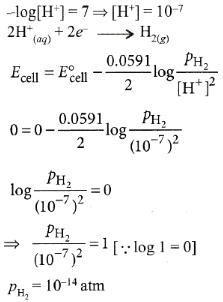Q.6. A device that converts energy of combustion of fuels like hydrogen and methane, directly into electrical energy is known as :    (2015)
A: Ni-Cd cell
B: Fuel Cell
C: Electrolytic Cell
D: Dynamo
Ans:
B
In fuel cell energy of combustion is converted into electrical energy.

Q.7. When 0.1 mol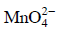is oxidised the quantity of electricity required to completely oxidise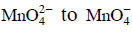is :    (2014)
A: 9650 C
B: 96.50 C
C: 96500 C
D: 2 × 96500 C
Ans: A

The oxidation reaction is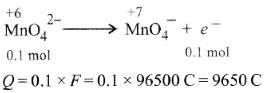Q.8. The weight of silver (at.wt. = 108) displaced by a quantity of electricity which displaces 5600 mL of O2 at STP will be :    (2014)
A: 54.0 g
B: 108.0 g
C: 5.4 g
D: 10.8 g
Ans:
B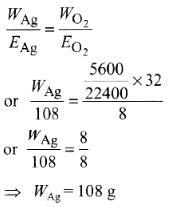Offer running on EduRev: Apply code STAYHOME200 to get INR 200 off on our premium plan EduRev Infinity!

,

,

,

,

,

,

,

,

,

,

,

,

,

,

,

,

,

,

,

,

,

;